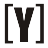# Kilograms (kg) to Pounds (lbs) Conversion

How to Convert Kilograms to Pounds (lbs): insert number in Kilograms box and press convert to conversion into Pounds (lbs).

Enter the number of kilograms and the tool will automatically convert it to pounds (lbs) for you.

kg

Pounds: lbs
Pounds+Ounces:
 lb
oz
Calculation:

## How to convert kg to pound (lbs)

1 kg = 2.20462262185 Pounds (lb)
1 lb = 0.45359237 kg

there are two ways to convert kilograms to pounds.
multiply the number of kilograms by 2.20462
or
divided the number of kilograms by 0.45359237
Formula kg to lbs
m (lb) = m (kg) / 0.45359237
The mass m in kilograms (kg) divided by 0.45359237
Alternative Formula kg to lbs
m (lb) = m (kg) x 2.20462
The mass m in kilograms (kg) multiply by 2.20462
Examples:
1. Convert 3 kg to lbs?
3 kg = 3 kg / 0.45359237 = 6.6138678655 lb
3 kg is 6.6138678655 pound.

or

2. Convert 5kg to lbs?
5 kilograms * 2.20462 = 11.023 pounds
So 5 kilograms is equal to 11.023 pounds.

## Difference Between Kilogram (kg) and Pounds (lbs)

Kilograms and pounds are both units of mass, but they are used in different measurement systems. The main difference between kilograms and pounds is the measurement system in which they are used.

kilogram (kg) VS Pounds (lbs)
A kilogram (kg) is a unit of mass in the International System of Units (SI). It is the base unit of mass in the SI system, and it is defined as the mass of a physical object, the international prototype of the kilogram, which is kept at the International Bureau of Weights and Measures in France.

Kilograms are used in the metric system, which is based on the decimal system and is used widely in science, medicine, and other fields.

Pounds (lbs) are a unit of mass used in the imperial system of measurement. One pound is equal to 0.45359237 kilograms.

Pounds are used in the imperial system, which is based on the British system of measurement and is used primarily in the United States.

### Kilograms (kg to lbs) Pounds Conversion Table

Kilograms (kg) Pounds (lb) Pounds+Ounces
(lb+oz)
0 kg 0 lb 0 lb 0 oz
0.1 kg 0.220 lb 0 lb 3.527 oz
1 kg 2.205 lb 2 lb 3.274 oz
2 kg 4.409 lb 4 lb 6.548 oz
3 kg 6.614 lb 6 lb 9.822 oz
4 kg 8.818 lb 8 lb 13.100 oz
5 kg 11.023 lb 11 lb 0.370 oz
6 kg 13.228 lb 13 lb 3.644 oz
7 kg 15.432 lb 15 lb 6.918 oz
8 kg 17.637 lb 17 lb 10.190 oz
9 kg 19.842 lb 19 lb 13.470 oz
10 kg 22.046 lb 22 lb 0.740 oz
20 kg 44.092 lb 44 lb 1.479 oz
30 kg 66.139 lb 66 lb 2.219 oz
40 kg 88.185 lb 88 lb 2.958 oz
50 kg 110.231 lb 110 lb 3.698 oz
60 kg 132.277 lb 132 lb 4.438 oz
70 kg 154.324 lb 154 lb 5.177 oz
80 kg 176.370 lb 176 lb 5.917oz
90 kg 198.416 lb 198 lb 6.657 oz
100 kg 220.462 lb 220 lb 7.396 oz
1000 kg 2204.623 lb 2204 lb 9.962 ozYoosFuhl.com Would you like to receive notifications on latest updates? No Yes